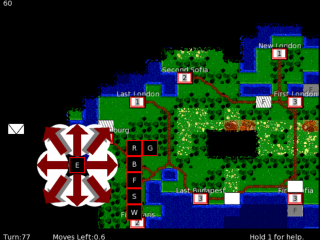-=+=- -=+=- -=+=- -=+=- -=+=- -=+=- -=+=- -=+=- -=+=- -=+=- -=+=- -=+=- -=+=- -=+=- -=+=- -=+=- -=+=- -=+=- -=+=- -=+=- -=+=- -=+=- -=+=- -=+=- -=+=- -=+=- -=+=- -=+=- -=+=- -=+=- (c) WidthPadding Industries 1987 0|89|0 -=+=- -=+=- -=+=- -=+=- -=+=- -=+=- -=+=- -=+=- -=+=- -=+=- -=+=- -=+=- -=+=- -=+=- -=+=- -=+=- -=+=- -=+=- -=+=- -=+=- -=+=- -=+=- -=+=- -=+=- -=+=- -=+=- -=+=- -=+=- -=+=- -=+=- SoCoder -> Showcase Home -> Strategy/RTS Games with characters that think a bit. Older -->A Civilization Clone v0.75 Browser based turn based strategy game Pakz , Monkey2 Browser Based GodOfMiniWars Hex&Chit Wargame PC gameleaper Blitz Max WarTactical WarTactical – A Turn Based Tactical War Game gameleaper HexWarrior HexWarrior – A Turn Based Strategic and Tactical Wargame gameleaper Blitz Max WindowsExtraterrestrial Grail Extraterrestrial Grail - The outer space board game of cunning and strategy! dantheman363 C/C++ WindowsBacterias WORK IN PROGRESS - Game similar to minions form CC martiinkoDomination Point Multiplayer only RTS Game where it's all about supplies. Spare C/C++ Windows Vesuvius online RTS massive war game mindstorm8191 Flash PSP VitaMushroom RTS Mushroom Buildings and Mushroom Men Fighting GoliX Blitz Windows Older -->